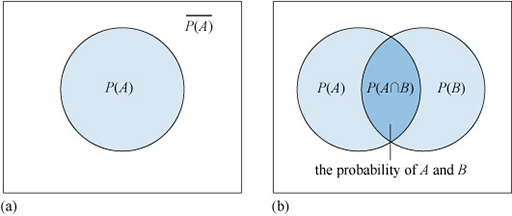### Become an OU studentAssessing risk in engineering, work and life

Start this free course now. Just create an account and sign in. Enrol and complete the course for a free statement of participation or digital badge if available.

# Combining probabilities

In risk assessments, it is rare to be concerned with the probability that a single event will happen or a single component will fail. As you saw in the Deepwater Horizon case study, several failures led to the incident. Therefore it is often necessary to look at the probability of combined events.

## Combining independent probabilities

For any event that has probability , the probability that does not occur is written as . If the probability is expressed as a fraction or a decimal then . This can be represented using a ‘probability space diagram’, as shown in Figure 15(a). The circle represents the probability that occurs, while the space outside the circle represents the probability that it does not occur.

If two events are independent (the outcome of one does not influence the outcome of the other), the probability of both events occurring is given by

For more than two events, the overall probability is given by multiplying the probabilities of each of the events expressed as a fraction or a decimal.

Using standard probability notation,

where the symbol means AND. This expression can be read as ‘the probability of and occurring is equal to the probability of occurring multiplied by the probability of occurring’. Again, this can be represented using a probability space diagram, as shown in Figure 15(b). Here there is one circle representing the probability of occurring and another representing the probability of occurring. The intersection of the two circles (shaded darker blue) represents .Figure 15 Probability space diagram for (a) ; (b)

Note that if two probabilities are dependent (the outcome of one does influence the outcome of the other), a different method has to be used to combine them, which is beyond the scope of this course. For example, suppose you draw two cards from a single pack of 52 playing cards – what is the probability that they will both be queens? The probability of the first card you draw being a queen is straightforward: there are four queens in a pack of 52 cards, so the probability is 4 in 52, or 1 in 13. However, when you draw the second card, the probability of drawing a queen will either be 3 in 51 (if the first card was a queen) or 4 in 51 (if the first card was not a queen). So the first event is affecting the outcome of the second – the events are dependent.